# braindecode.models.ShallowFBCSPNet#

class braindecode.models.ShallowFBCSPNet(in_chans, n_classes, input_window_samples=None, n_filters_time=40, filter_time_length=25, n_filters_spat=40, pool_time_length=75, pool_time_stride=15, final_conv_length=30, conv_nonlin=<function square>, pool_mode='mean', pool_nonlin=<function safe_log>, split_first_layer=True, batch_norm=True, batch_norm_alpha=0.1, drop_prob=0.5)[source]#

Shallow ConvNet model from Schirrmeister et al 2017.

Model described in [Schirrmeister2017].

Parameters
• in_chans (int) – Number of EEG input channels.

• n_classes (int) – Number of classes to predict (number of output filters of last layer).

• input_window_samples (int | None) – Only used to determine the length of the last convolutional kernel if final_conv_length is “auto”.

• n_filters_time (int) – Number of temporal filters.

• filter_time_length (int) – Length of the temporal filter.

• n_filters_spat (int) – Number of spatial filters.

• pool_time_length (int) – Length of temporal pooling filter.

• pool_time_stride (int) – Length of stride between temporal pooling filters.

• final_conv_length (int | str) – Length of the final convolution layer. If set to “auto”, input_window_samples must not be None.

• conv_nonlin (callable) – Non-linear function to be used after convolution layers.

• pool_mode (str) – Method to use on pooling layers. “max” or “mean”.

• pool_nonlin (callable) – Non-linear function to be used after pooling layers.

• split_first_layer (bool) – Split first layer into temporal and spatial layers (True) or just use temporal (False). There would be no non-linearity between the split layers.

• batch_norm (bool) – Whether to use batch normalisation.

• batch_norm_alpha (float) – Momentum for BatchNorm2d.

• drop_prob (float) – Dropout probability.

References

Schirrmeister2017

Schirrmeister, R. T., Springenberg, J. T., Fiederer, L. D. J., Glasstetter, M., Eggensperger, K., Tangermann, M., Hutter, F. & Ball, T. (2017). Deep learning with convolutional neural networks for EEG decoding and visualization. Human Brain Mapping , Aug. 2017. Online: http://dx.doi.org/10.1002/hbm.23730

## Examples using `braindecode.models.ShallowFBCSPNet`#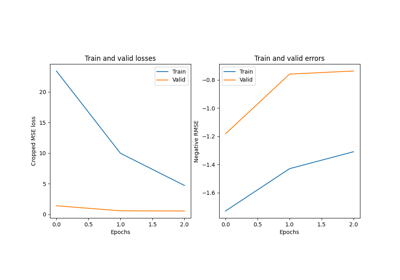Regression example on fake data

Regression example on fake data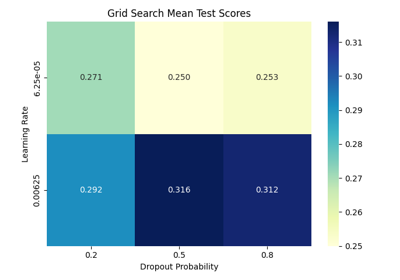Hyperparameter tuning with scikit-learn

Hyperparameter tuning with scikit-learn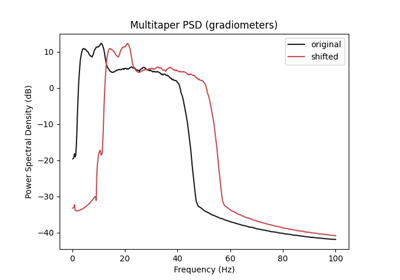Data Augmentation on BCIC IV 2a Dataset

Data Augmentation on BCIC IV 2a Dataset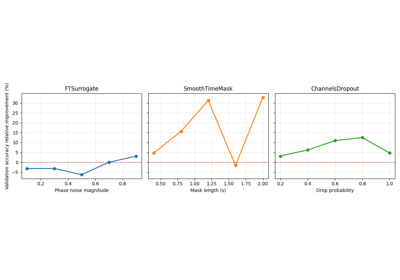Searching the best data augmentation on BCIC IV 2a Dataset

Searching the best data augmentation on BCIC IV 2a Dataset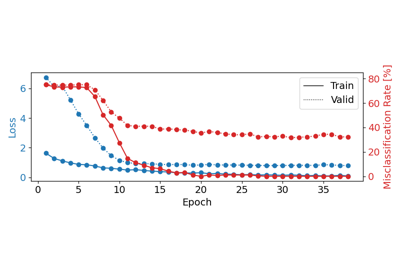Trialwise Decoding on BCIC IV 2a Dataset

Trialwise Decoding on BCIC IV 2a DatasetFingers flexion decoding on BCIC IV 4 ECoG Dataset

Fingers flexion decoding on BCIC IV 4 ECoG Dataset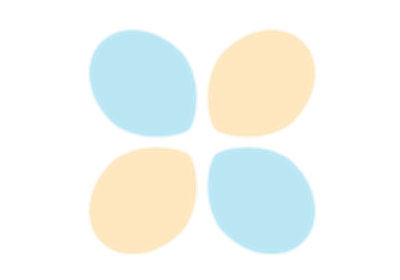Fingers flexion cropped decoding on BCIC IV 4 ECoG Dataset

Fingers flexion cropped decoding on BCIC IV 4 ECoG Dataset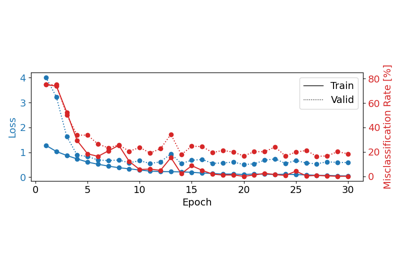Cropped Decoding on BCIC IV 2a Dataset

Cropped Decoding on BCIC IV 2a Dataset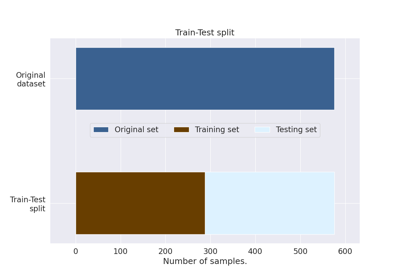How to train, test and tune your model

How to train, test and tune your model